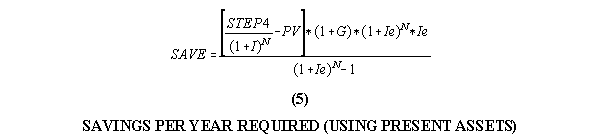Retirement Formula

March 17, 1975

Derived by Bill Larsen

1 Determine the retirement needs per year in current dollars.

2 Determine the following variables:

2.1 Rate of return assets will earn I = __________

2.2 Inflation rate G = __________

2.3 Years till retirement N = __________

2.4 Number of years in retirement X = __________

2.5 Present value of retirement assets PV= __________

3 Calculate future value of retirement needs per year.

FVneeds= (STEP 1) * (1+G)N

Future value of needs at retirement

4 Calculate future value of assets required to sustain retirement needs in step 3.

Ie = (1+I)/(1+G)-1

Effective Rate of Return

FVassets =(STEP 3)*((1+Ie)X-1)/((1+Ie)X *(1+G)*Ie)

Future value of assets needed.

5 Calculate the amount to save each year to reach goal in step 4. Increase amount saved each year by inflation or own goal. NOTE: If other than inflation a new effective rate must be calculated for this step only.

SAVEyear=((STEP 4)/(1+I)N))*(1+G)*(1+Ie)N *Ie/((1+Ie)N-1)

IF YOU HAVE PRESENT ASSETS

SAVEyear=((STEP 4)/(1+I)N-PV))*(1+G)*(1+Ie)N * Ie/((1+Ie)N-1)

6 Recalculate each year up till retirement to minimize any large fluctuations in rate of return, inflation, savings rate and retirement age.

7 During retirement recalculate yearly disbursements based on the previous years variables determined in step 2. Note: X will decrease each year you are retired.

DISBURSEMENT= (ASSETS*(1+G)*(1+Ie)X * Ie)/((1+Ie)X-1)

PICTORIAL CORRECT FORMULAS